This site uses cookies. By continuing to browse the ConceptDraw site you are agreeing to our Use of Site Cookies.
ConceptDraw Samples | Computer and networks — Wireless network diagrams

# Wireless Networks

Samples of wireless network diagrams are created using ConceptDraw DIAGRAM diagramming and vector drawing software enhanced with solution Wireless Networks from ConceptDraw Solution Park.

ConceptDraw DIAGRAM provides export of vector graphic multipage documents into multiple file formats: vector graphics (SVG, EMF, EPS), bitmap graphics (PNG, JPEG, GIF, BMP, TIFF), web documents (HTML, PDF), PowerPoint presentations (PPT), Adobe Flash (SWF).

## Tutorials and Solutions:

Video Tutorials: ConceptDraw Solution Park

Solutions: Wireless Networks for ConceptDraw DIAGRAM

## Sample 1: Global Positioning System (GPS) Operation

Wireless Network Diagram sample: Global positioning system (GPS) operation.

This example is created using ConceptDraw DIAGRAM diagramming and vector drawing software enhanced with Wireless Network solution from ConceptDraw Solution Park.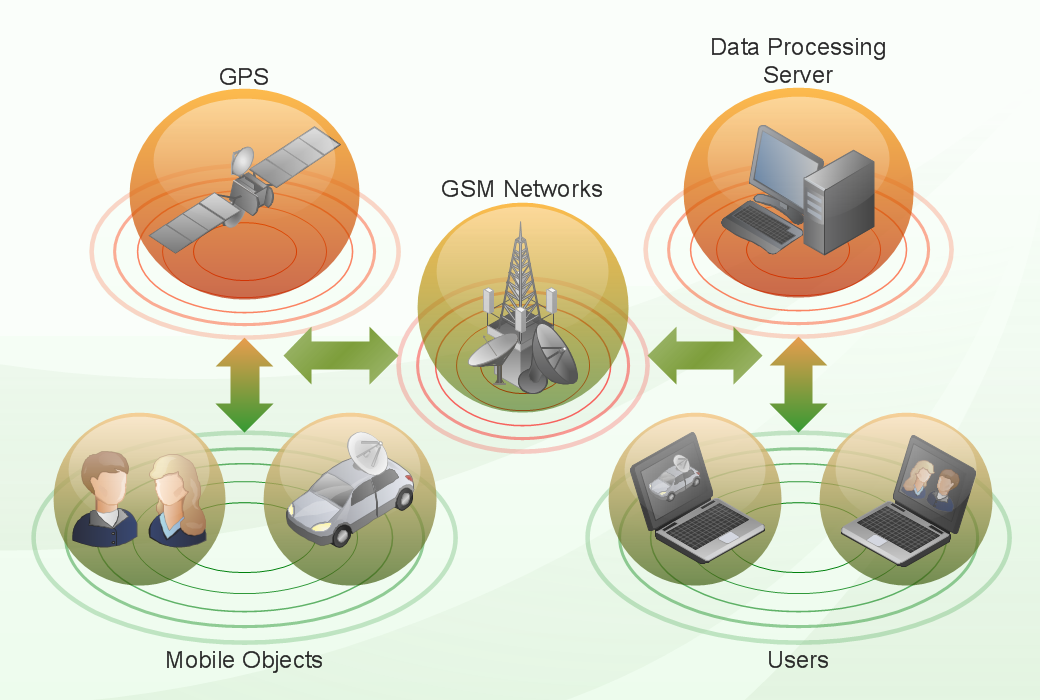## Sample 2: Satellite

Wireless Network Diagram sample: Satellite.

This example is created using ConceptDraw DIAGRAM diagramming and vector drawing software enhanced with Wireless Network solution from ConceptDraw Solution Park.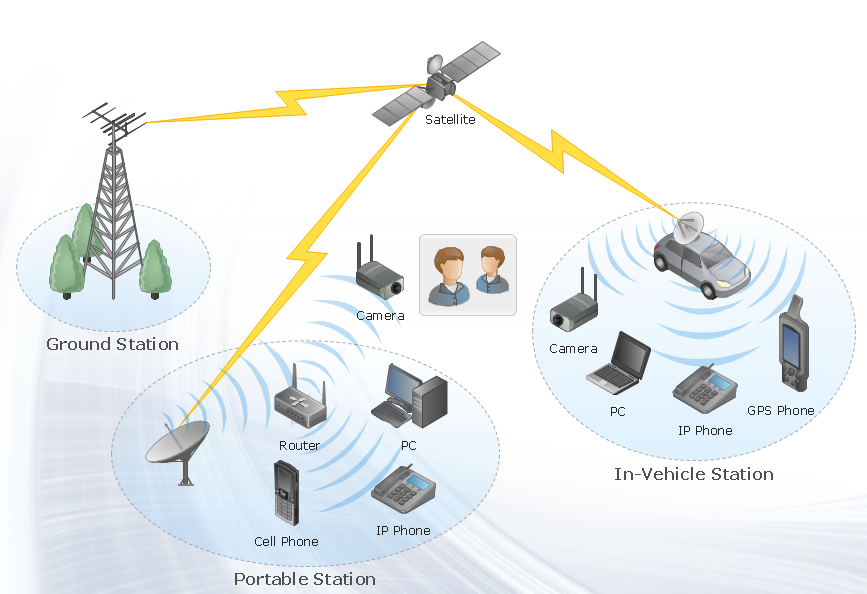## Sample 3: Ultra High Performance WLANs

Wireless Network Diagram sample: Ultra high performance WLANs.

This example is created using ConceptDraw DIAGRAM diagramming and vector drawing software enhanced with Wireless Network solution from ConceptDraw Solution Park.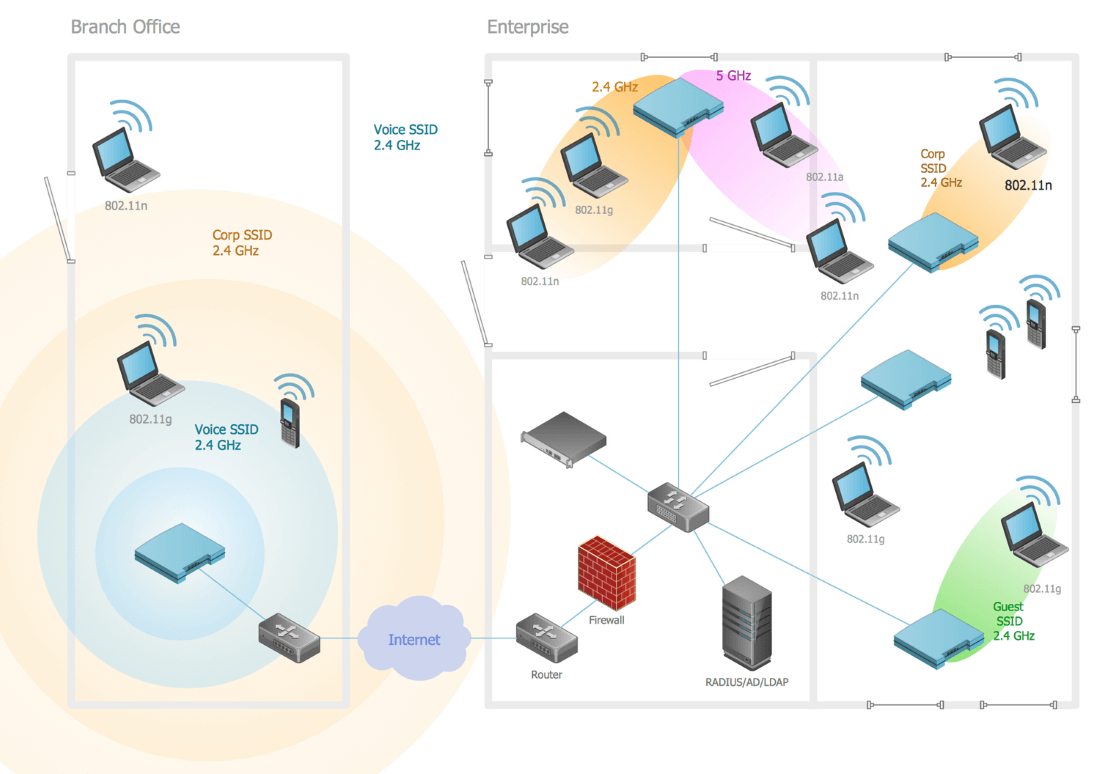This example is created using ConceptDraw DIAGRAM diagramming and vector drawing software enhanced with Wireless Network solution from ConceptDraw Solution Park.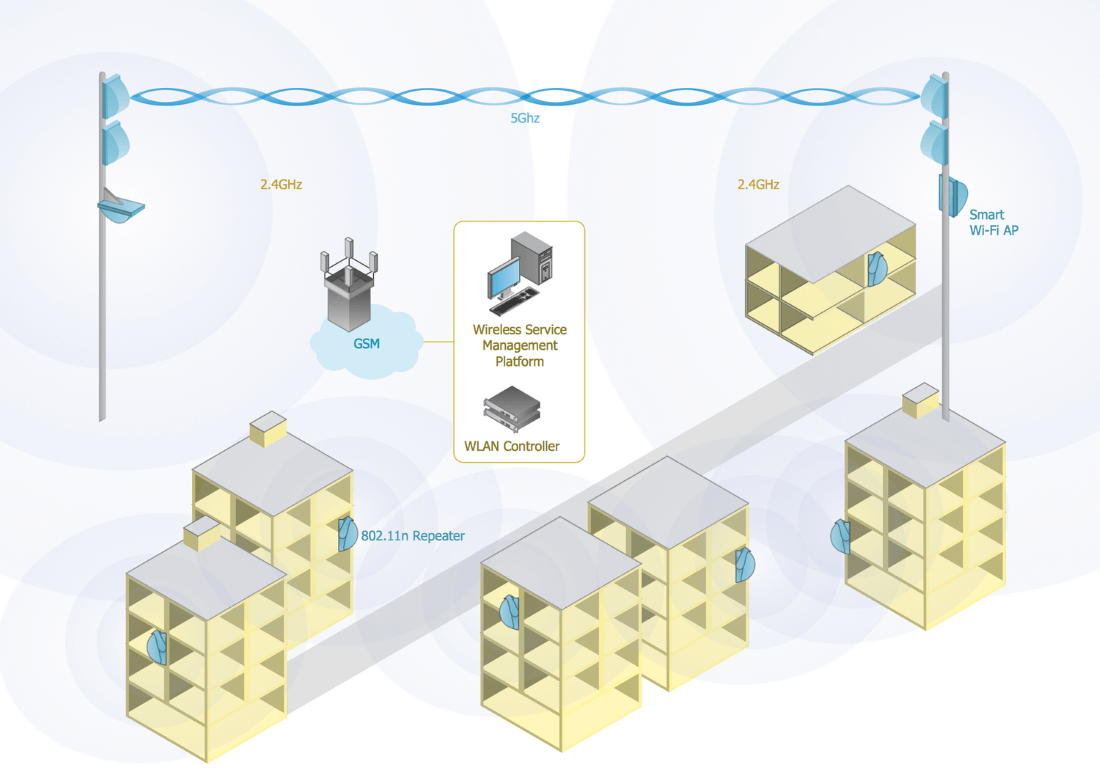## Sample 5: Wireless Access Point

Wireless Network Diagram sample: Wireless access point.

This example is created using ConceptDraw DIAGRAM diagramming and vector drawing software enhanced with Wireless Network solution from ConceptDraw Solution Park.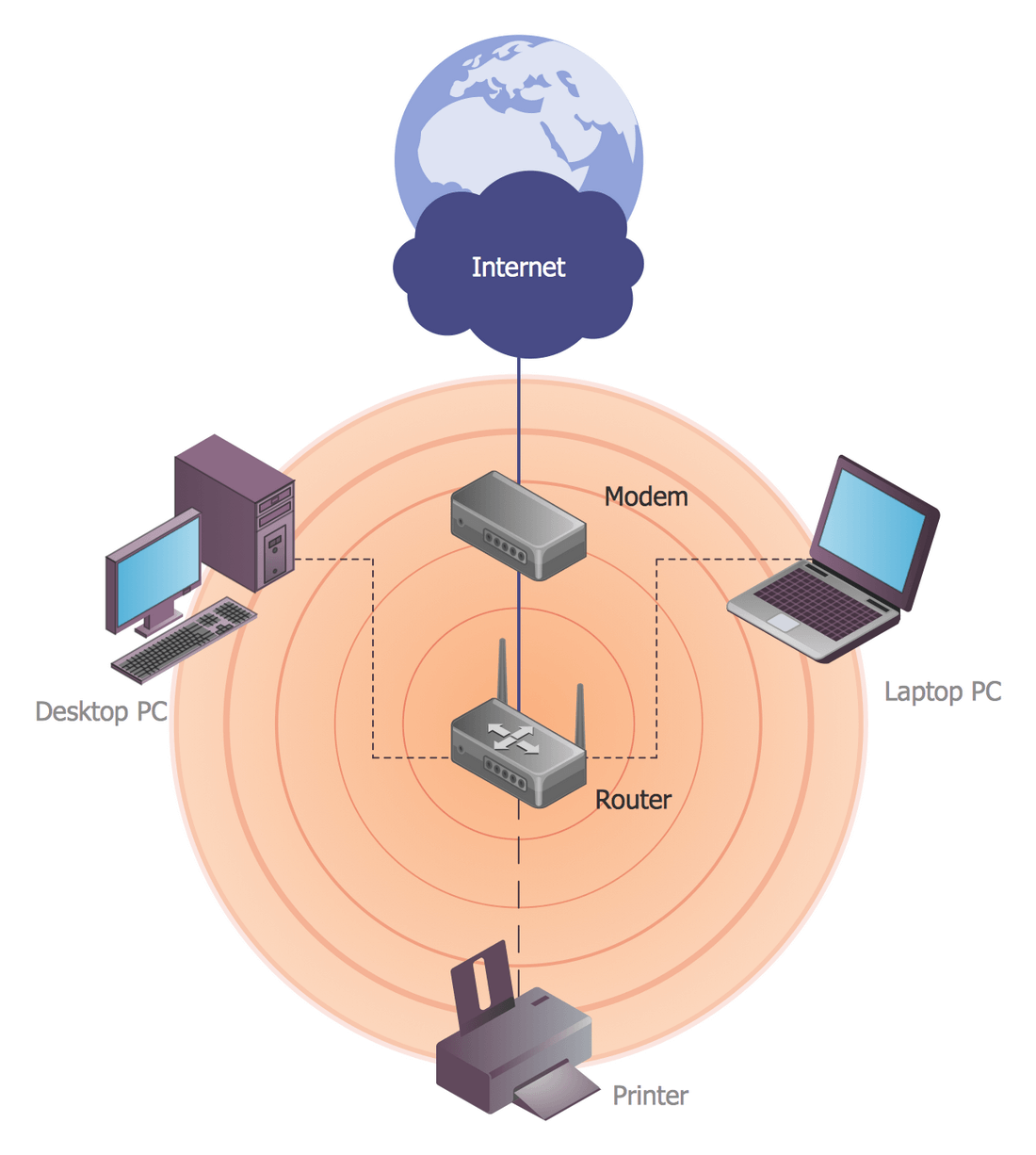## Sample 6: Wireless Mesh Network Diagram

Wireless Network Diagram sample: Wireless mesh network.

This example is created using ConceptDraw DIAGRAM diagramming and vector drawing software enhanced with Wireless Network solution from ConceptDraw Solution Park.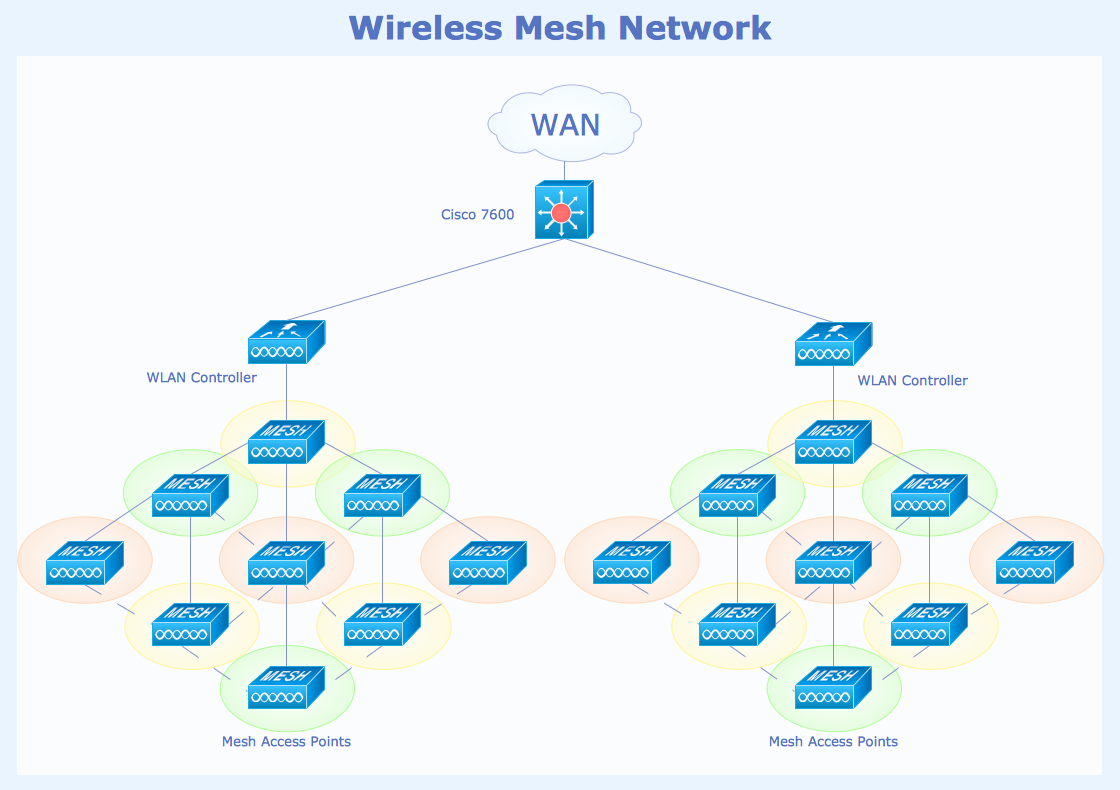## Sample 7: Wireless Broadband Network Layout Diagram

Wireless Network Diagram sample: Wireless broadband network layout.

This example is created using ConceptDraw DIAGRAM diagramming and vector drawing software enhanced with Wireless Network solution from ConceptDraw Solution Park.## Sample 8: Roaming Wireless Local Area Network Diagram

Wireless Network Diagram sample: Roaming wireless local area network.

This example is created using ConceptDraw DIAGRAM diagramming and vector drawing software enhanced with Wireless Network solution from ConceptDraw Solution Park.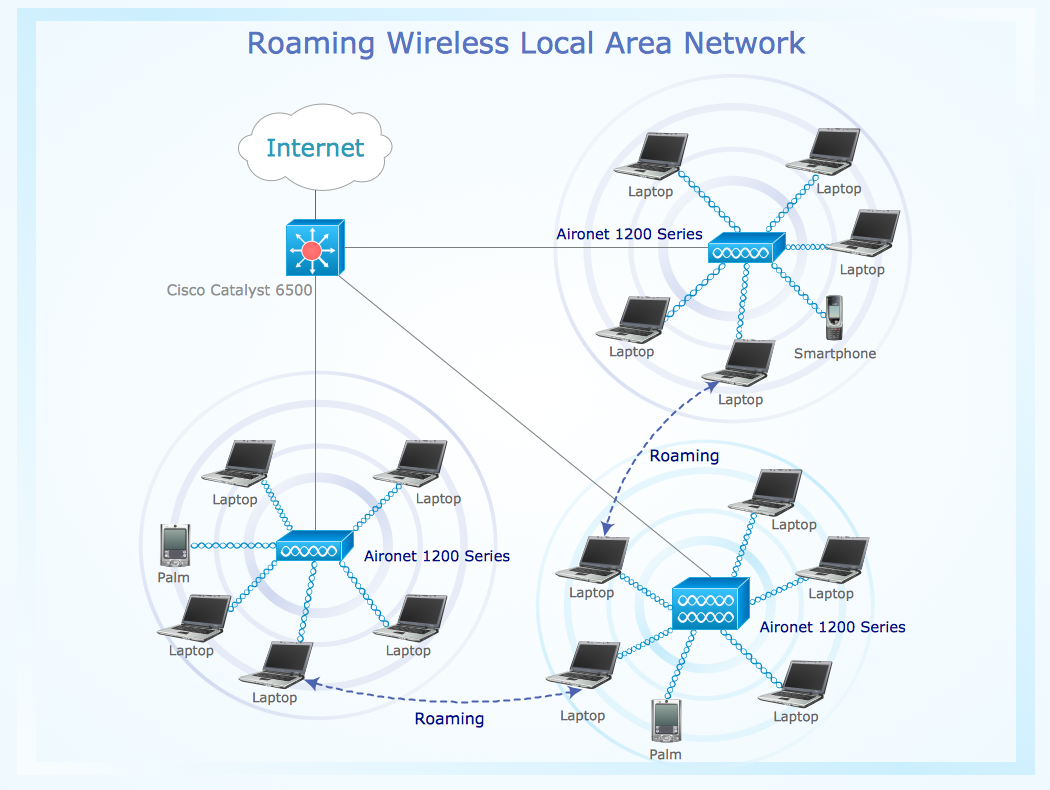## Sample 9: Home Wireless Network

Wireless Network Diagram sample: Home wireless network.

This example is created using ConceptDraw DIAGRAM diagramming and vector drawing software enhanced with Wireless Network solution from ConceptDraw Solution Park.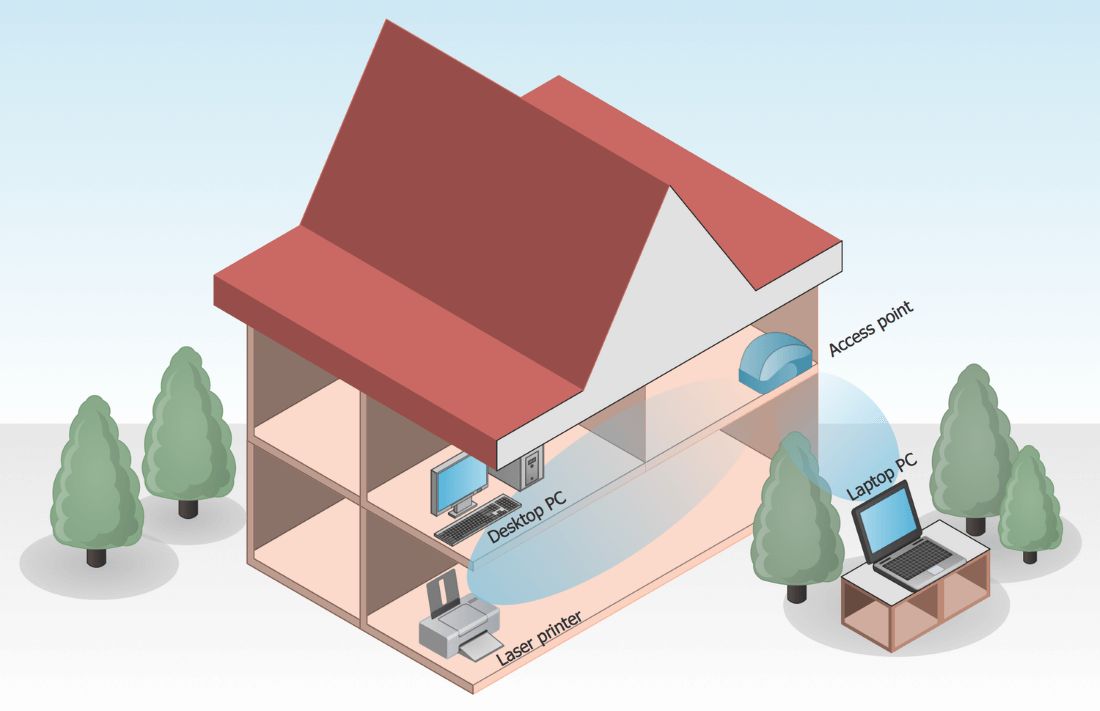## Sample 10: Long-Range Wi-Fi

Wireless Network Diagram sample: Long-range Wi-Fi.

This example is created using ConceptDraw DIAGRAM diagramming and vector drawing software enhanced with Wireless Network solution from ConceptDraw Solution Park.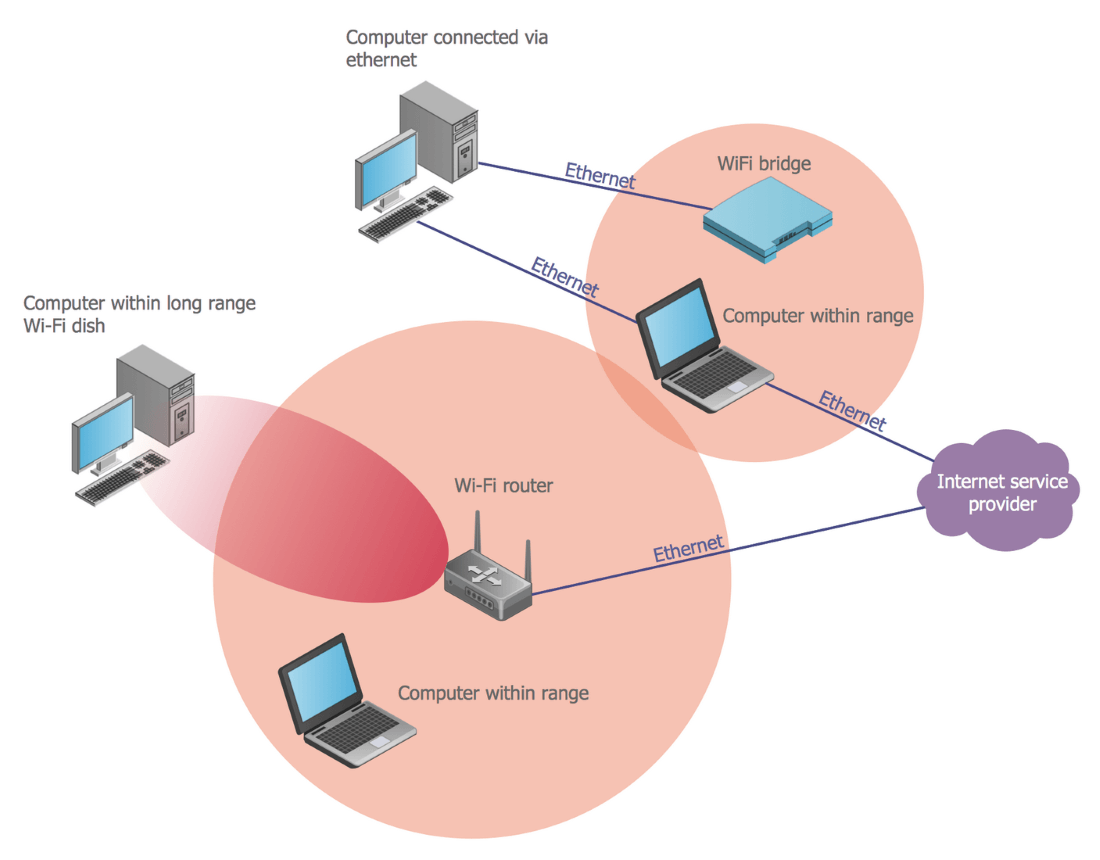## Sample 11: Enterprise WMN

Wireless Network Diagram sample: Enterprise WMN.

This example is created using ConceptDraw DIAGRAM diagramming and vector drawing software enhanced with Wireless Network solution from ConceptDraw Solution Park.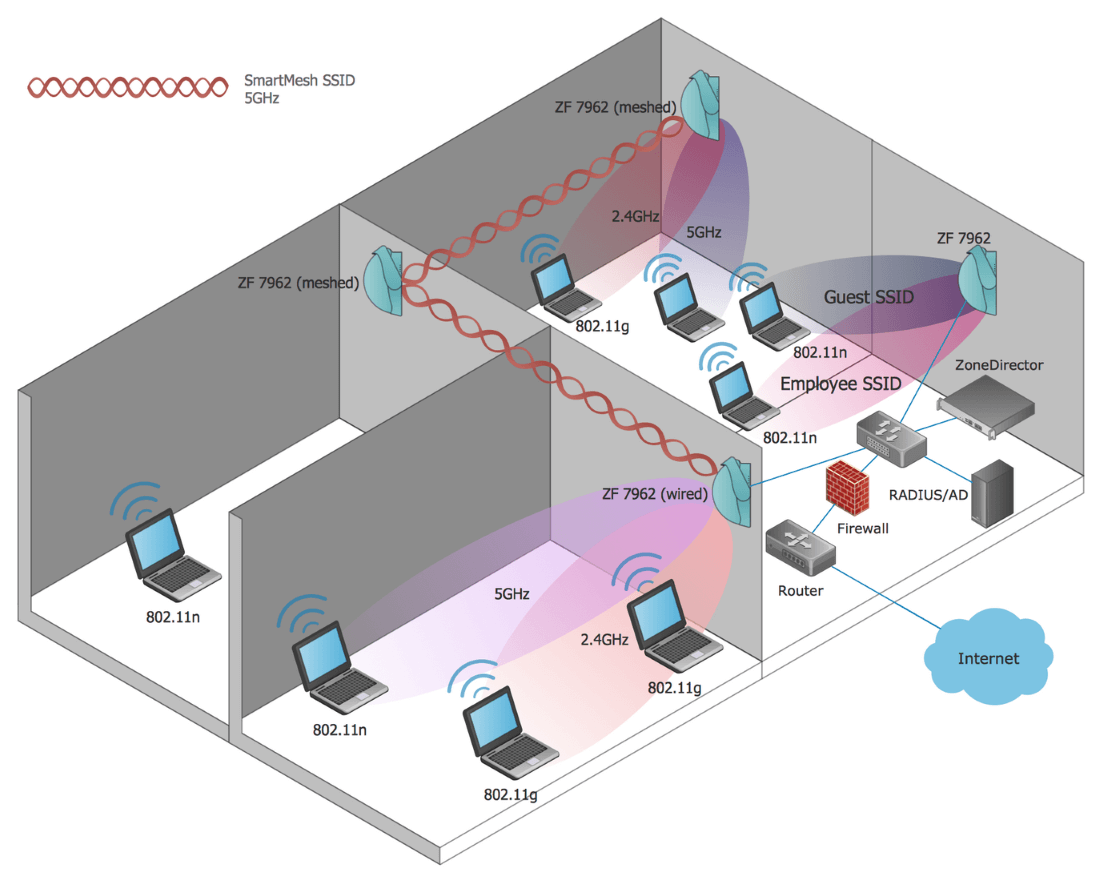## Sample 12: GSM Backhaul

Wireless Network Diagram sample: GSM Backhaul.

This example is created using ConceptDraw DIAGRAM diagramming and vector drawing software enhanced with Wireless Network solution from ConceptDraw Solution Park.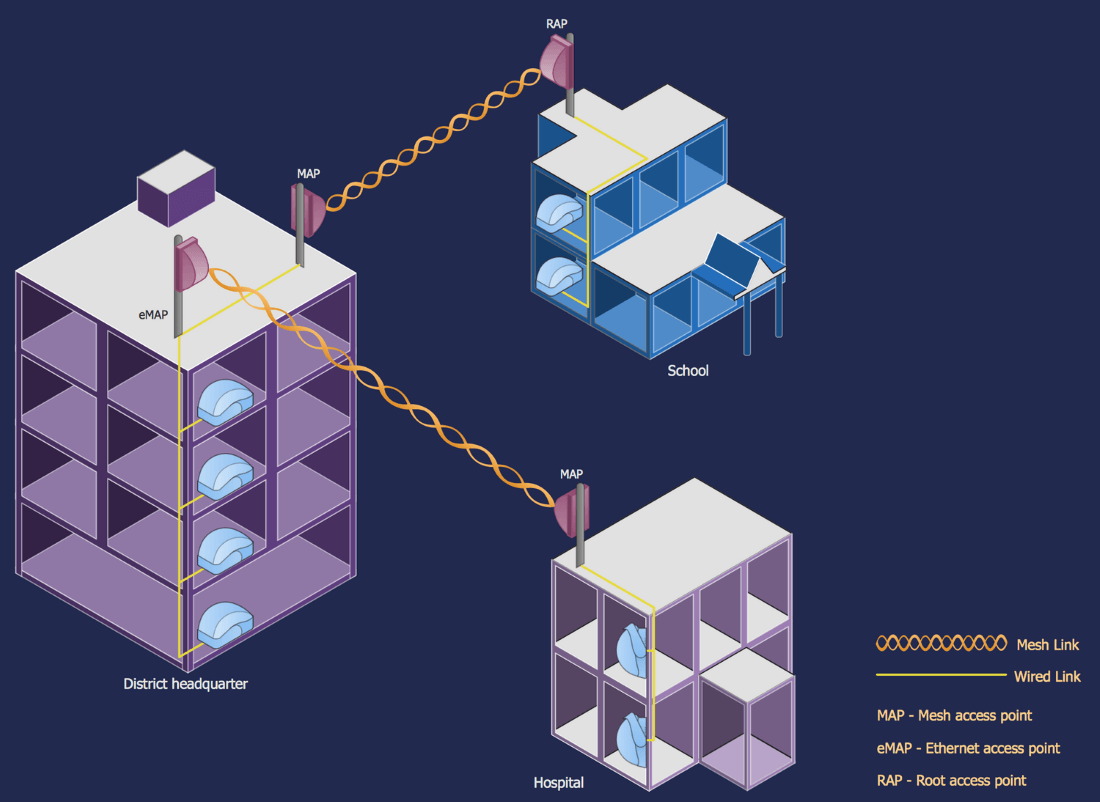## Sample 13: Office WLAN

Wireless Network Diagram sample: Office WLAN.

This example is created using ConceptDraw DIAGRAM diagramming and vector drawing software enhanced with Wireless Network solution from ConceptDraw Solution Park.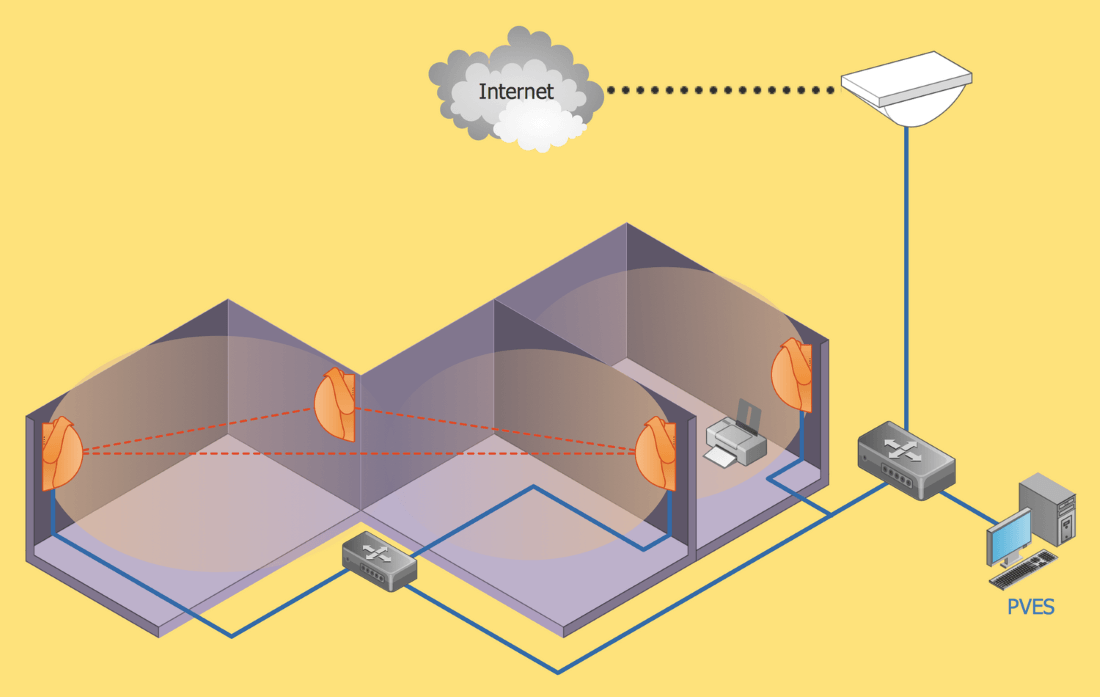## Sample 14: Secure Wireless Networking

Wireless Network Diagram sample: Secure Wireless Networking.

This example is created using ConceptDraw DIAGRAM diagramming and vector drawing software enhanced with Wireless Network solution from ConceptDraw Solution Park.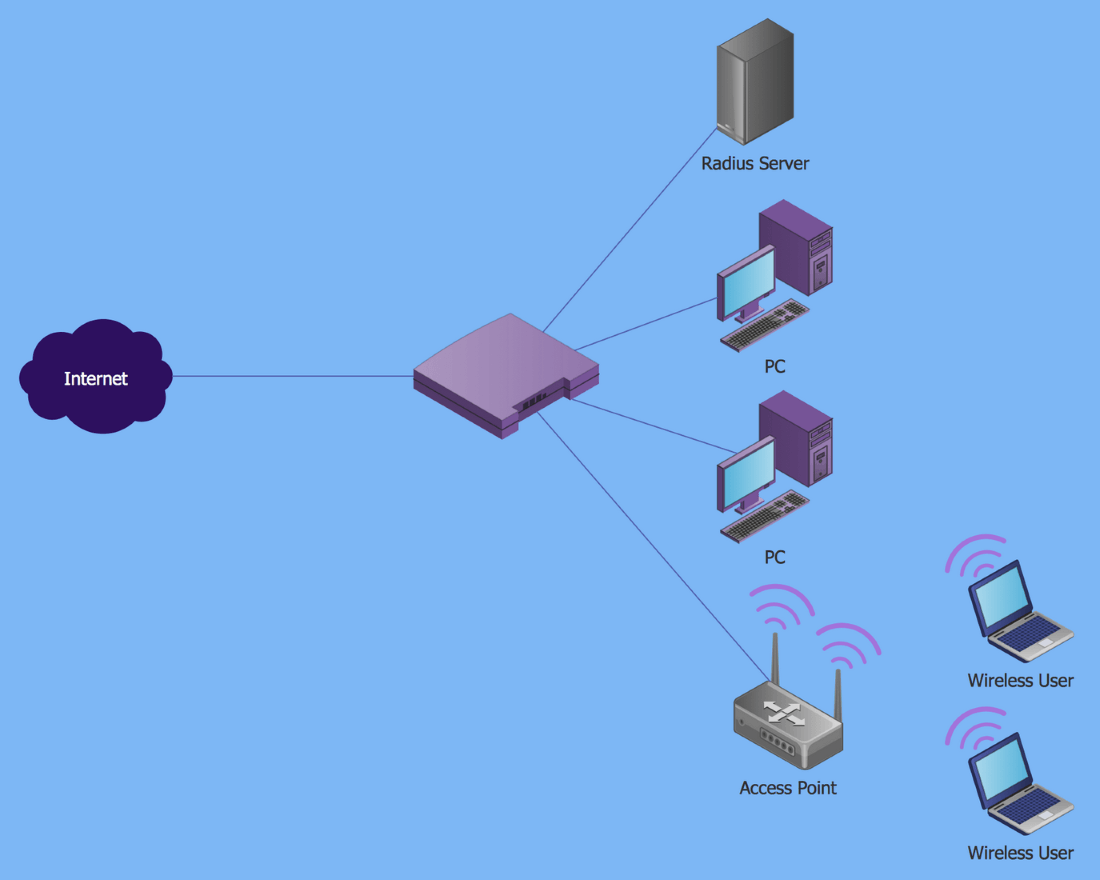## Sample 15: Wi-Fi Repeater

Wireless Network Diagram sample: Wi-Fi Repeater.

This example is created using ConceptDraw DIAGRAM diagramming and vector drawing software enhanced with Wireless Network solution from ConceptDraw Solution Park.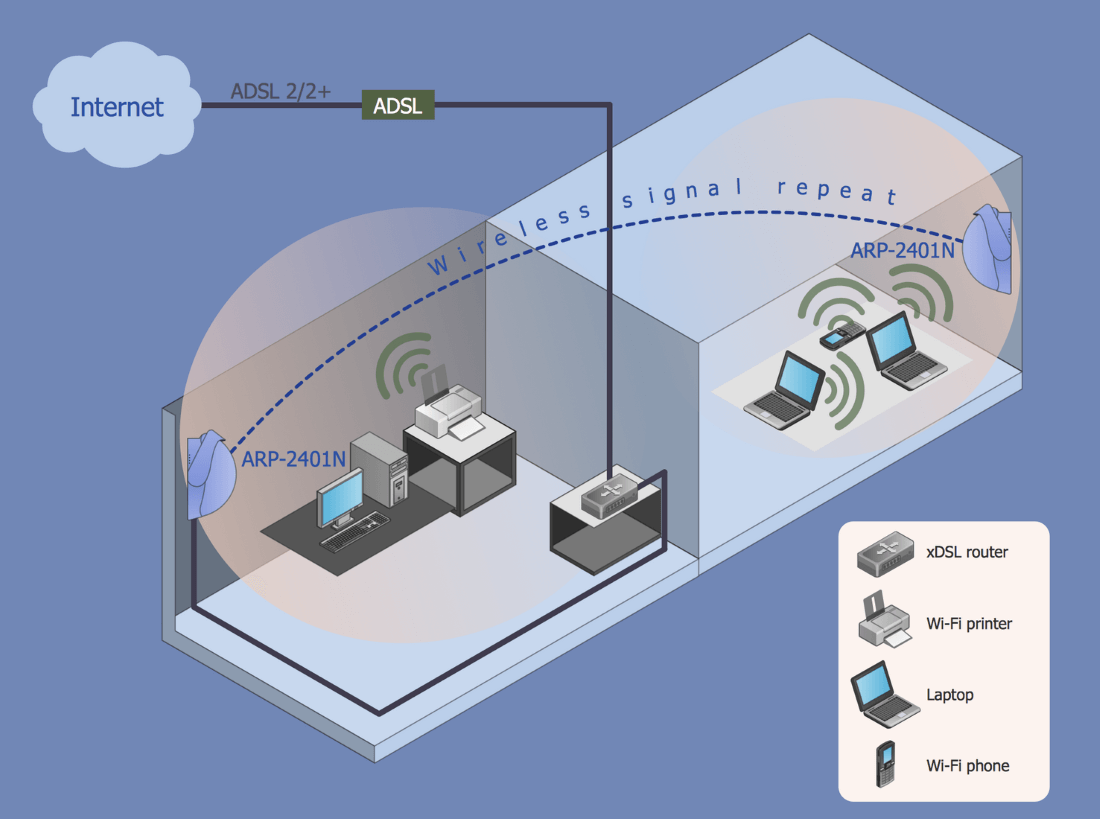All samples are copyrighted CS Odessa's.

Usage of them is covered by Creative Commons “Attribution Non-Commercial No Derivatives” License.

The text you can find at: https://creativecommons.org/licenses/by-nc-nd/3.0